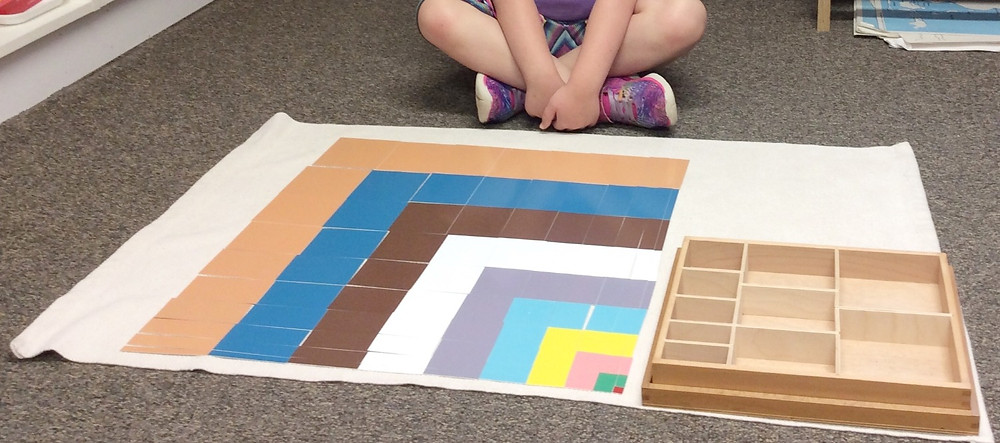CATEGORIES

# Montessori Monday: The Square of the DecanomialSome of our favorite Montessori materials are the ones that make elegant and simple seemingly complicated concepts. The math materials are especially good at this, translating concepts you may have learned (or misunderstood!) in high school into engaging puzzles appropriate for young children to explore. The Square of the Decanomial is one such material. Beautiful and orderly, this material makes concrete the relationships of multiplied squares for as many as ten variables. Yes, you read that right: children in Montessori early childhood classrooms are exploring the relationships of equations as complicated as (a+b+c+d+e+f+g+h+i+j)((a+b+c+d+e+f+g+h+i+j). The presentation can be introduced either numerically, algebraically or geometrically. In its initial presentation, the concepts can be introduced through squares and chains similar to those in the chain cabinet, allowing the children to build out larger and larger squares on his or her mat by fitting together combinations of beads. An equation including two squares, for example, or (a+b)(a+b), which results in the quotient a2+2ab+b2, will require two different size squares (one for a and one for b) and two chains the length of a and the height of b. Placed together, these four chain segments will construct one larger square, the square of the binomial. Use three variables and you can build the square of the trinomial. four for the quatrenomial, five for the pentenomial, and so on. Here, notice that the child is exploring these concepts with a prepared set of rectangles and squares, each representing the same quantities she may first have explored in the beads. When she completes laying out the lilac rectangles, she will have crafted the larger square of (a+b+c+d+e+f)(a+b+c+d+e+f). Not bad for a morning's work! Children who understand these relationships in quantities (where b = 2 and c = 3, for example) can puzzle out how to write the relationships they observe in variables. Ultimately, they come to understand the parts of all those extended algebraic formulas, not because they've memorized a pattern to get the right answer, but because they understand what a square is and how combining multiple squares also requires combinations of rectangles if you want to create an orderly solution. Children exploring this material for the first time may engage with it as a pattern of geometric relationships rather than for its numeric or algebraic qualities. That's ok. By making these relationships concrete and malleable, the Montessori materials provide a hands-on concrete knowledge of abstract concepts, allowing the child to better understand those concepts, whether they encounter them this year, next or ten years from now in Sophomore Algebra class.